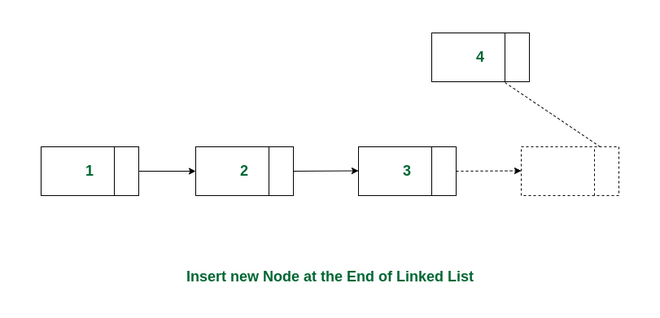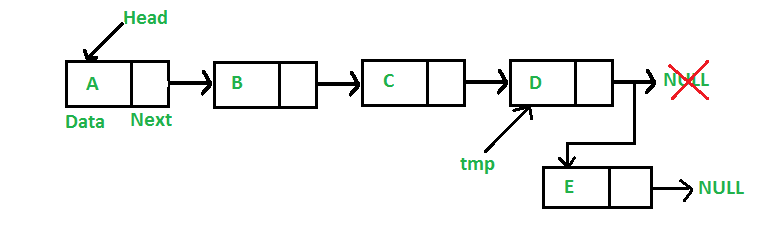Open in App
Not now

# Insert Node at the End of a Linked List

• Difficulty Level : Basic
• Last Updated : 13 Mar, 2023

Like arrays, Linked List is a linear data structure. Unlike arrays, linked list elements are not stored at a contiguous location; the elements are linked using pointers. They include a series of connected nodes. Here, each node stores the data and the address of the next node.

Given a linked list, the task is to insert a new node at the end of the linked list.Insert a node at the end of a linked list

Example:

List = 1->2->3->4, Insert a node with value 5 at the end.
Output list will be: 1->2->3->4->5

Consider the following representations of the linked list.

## C++

 `// A linked list node` `class` `Node {` `public``:` `    ``int` `data;` `    ``Node* next;` `};`

## C

 `// A linked list node` `struct` `Node {` `    ``int` `data;` `    ``struct` `Node* next;` `};`

## Java

 `// Linked List Class` `class` `LinkedList {` `    ``Node head; ``// head of list`   `    ``/* Node Class */` `    ``class` `Node {` `        ``int` `data;` `        ``Node next;`   `        ``// Constructor to create a new node` `        ``Node(``int` `d)` `        ``{` `            ``data = d;` `            ``next = ``null``;` `        ``}` `    ``}` `}`

## Python3

 `# Node class` `class` `Node:`   `    ``# Function to initialize the node object` `    ``def` `__init__(``self``, data):` `        ``self``.data ``=` `data  ``# Assign data` `        ``self``.``next` `=` `None`  `# Initialize next as null`   `# Linked List class`     `class` `LinkedList:`   `    ``# Function to initialize the Linked List object` `    ``def` `__init__(``self``):` `        ``self``.head ``=` `None`

## C#

 `/* Linked list Node*/` `public` `class` `Node {` `    ``public` `int` `data;` `    ``public` `Node next;` `    ``public` `Node(``int` `d)` `    ``{` `        ``data = d;` `        ``next = ``null``;` `    ``}` `}`

## Javascript

 ``

Approach: Following is the approach to add a new node at the end of the linked list:

• Allocate memory for a new node (say temp) and create a pointer (say last) which points the head of the linked list.
• Set the data to be entered in temp.
• temp will be the last node. Hence the next pointer of temp will point to null.
• Traverse using the last pointer till we reach the end node of the linked list.
• Now, point the next node of last to temp.

Follow the below image for a better understanding:How to insert new node at the end of the linked list

Below is the implementation of the approach:

## C++

 `// Given a reference (pointer to pointer) to the head` `// of a list and an int, appends a new node at the end` `void` `append(Node** head_ref, ``int` `new_data)` `{` `    ``// 1. allocate node` `    ``Node* new_node = ``new` `Node();`   `    ``// Used in step 5` `    ``Node* last = *head_ref;`   `    ``// 2. Put in the data` `    ``new_node->data = new_data;`   `    ``// 3. This new node is going to be` `    ``// the last node, so make next of` `    ``// it as NULL` `    ``new_node->next = NULL;`   `    ``// 4. If the Linked List is empty,` `    ``// then make the new node as head` `    ``if` `(*head_ref == NULL) {` `        ``*head_ref = new_node;` `        ``return``;` `    ``}`   `    ``// 5. Else traverse till the last node` `    ``while` `(last->next != NULL) {` `        ``last = last->next;` `    ``}`   `    ``// 6. Change the next of last node` `    ``last->next = new_node;` `    ``return``;` `}`

## C

 `/* Given a reference (pointer to pointer) to the head` `   ``of a list and an int, appends a new node at the end  */` `void` `append(``struct` `Node** head_ref, ``int` `new_data)` `{` `    ``/* 1. allocate node */` `    ``struct` `Node* new_node` `        ``= (``struct` `Node*)``malloc``(``sizeof``(``struct` `Node));`   `    ``struct` `Node* last = *head_ref; ``/* used in step 5*/`   `    ``/* 2. put in the data  */` `    ``new_node->data = new_data;`   `    ``/* 3. This new node is going to be the last node, so` `       ``make next of it as NULL*/` `    ``new_node->next = NULL;`   `    ``/* 4. If the Linked List is empty, then make the new` `     ``* node as head */` `    ``if` `(*head_ref == NULL) {` `        ``*head_ref = new_node;` `        ``return``;` `    ``}`   `    ``/* 5. Else traverse till the last node */` `    ``while` `(last->next != NULL)` `        ``last = last->next;`   `    ``/* 6. Change the next of last node */` `    ``last->next = new_node;` `    ``return``;` `}`

## Java

 `/* Appends a new node at the end.  This method is` `   ``defined inside LinkedList class shown above */` `public` `void` `append(``int` `new_data)` `{` `    ``/* 1. Allocate the Node &` `       ``2. Put in the data` `       ``3. Set next as null */` `    ``Node new_node = ``new` `Node(new_data);`   `    ``/* 4. If the Linked List is empty, then make the` `           ``new node as head */` `    ``if` `(head == ``null``) {` `        ``head = ``new` `Node(new_data);` `        ``return``;` `    ``}`   `    ``/* 4. This new node is going to be the last node, so` `         ``make next of it as null */` `    ``new_node.next = ``null``;`   `    ``/* 5. Else traverse till the last node */` `    ``Node last = head;` `    ``while` `(last.next != ``null``)` `        ``last = last.next;`   `    ``/* 6. Change the next of last node */` `    ``last.next = new_node;` `    ``return``;` `}`

## Python3

 `# This function is defined in Linked List class` `# Appends a new node at the end.  This method is` `# defined inside LinkedList class shown above` `def` `append(``self``, new_data):`   `        ``# 1. Create a new node` `        ``# 2. Put in the data` `        ``# 3. Set next as None` `        ``new_node ``=` `Node(new_data)`   `        ``# 4. If the Linked List is empty, then make the` `        ``# new node as head` `        ``if` `self``.head ``is` `None``:` `            ``self``.head ``=` `new_node` `            ``return`   `        ``# 5. Else traverse till the last node` `        ``last ``=` `self``.head` `        ``while` `(last.``next``):` `            ``last ``=` `last.``next`   `        ``# 6. Change the next of last node` `        ``last.``next` `=` `new_node`

## C#

 `/* Appends a new node at the end. This method is` `defined inside LinkedList class shown above */` `public` `void` `append(``int` `new_data)` `{` `    ``/* 1. Allocate the Node &` `    ``2. Put in the data` `    ``3. Set next as null */` `    ``Node new_node = ``new` `Node(new_data);`   `    ``/* 4. If the Linked List is empty,` `       ``then make the new node as head */` `    ``if` `(head == ``null``) {` `        ``head = ``new` `Node(new_data);` `        ``return``;` `    ``}`   `    ``/* 4. This new node is going to be` `    ``the last node, so make next of it as null */` `    ``new_node.next = ``null``;`   `    ``/* 5. Else traverse till the last node */` `    ``Node last = head;` `    ``while` `(last.next != ``null``)` `        ``last = last.next;`   `    ``/* 6. Change the next of last node */` `    ``last.next = new_node;` `    ``return``;` `}`

## Javascript

 ``

Time Complexity: O(N) where N is the length of the linked list
Auxiliary Space: O(1)

My Personal Notes arrow_drop_up
Related Articles